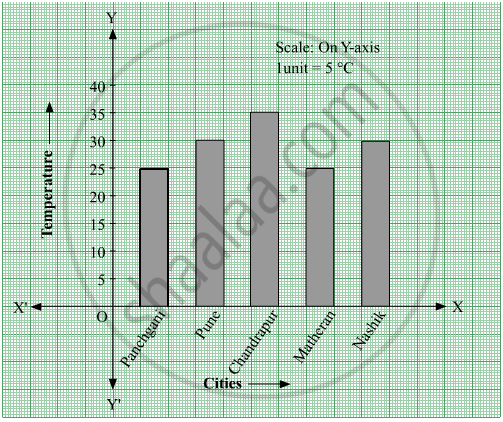# This Bar Graph Shows the Maximum Temperatures in Degrees Celsius in Different Cities on a Certain Day in February. Observe the Graph and Answer the Questions. - Mathematics

Graph

This bar graph shows the maximum temperatures in degrees Celsius in different cities on a certain day in February. Observe the graph and answer the questions.1. What data is shown on the vertical and the horizontal lines?
2. Which city had the highest temperature?
3. Which cities had equal maximum temperatures?
4. Which cities had a maximum temperature of 30°C?
5. What is the difference between the maximum temperatures of Panchgani and Chandrapur?

#### Solution

1. Temperature is shown on the vertical line (Y-axis) and different cities are shown on the horizontal line (X-axis).
2. Chandrapur had the highest temperature (35°C) as the height of the bar corresponding to the city Chandrapur is maximum.
3. Panchgani and Matheran had an equal maximum temperature of 25°C. Also, Pune and Nasik had an equal maximum temperature of 30°C. Thus, the cities Panchgani and Matheran; Pune and Nasik had equal maximum temperatures.
4. Pune and Nasik had a maximum temperature of 30°C.
5. Maximum temperature of Panchgani = 25°C
Maximum temperature of Chandrapur = 35°C
∴ Difference between the maximum temperatures of Panchgani and Chandrapur = 35°C − 25°C = 10°C
Is there an error in this question or solution?

#### APPEARS IN

Balbharati Mathematics 6th Standard Maharashtra State Board
Chapter 6 Bar Graphs
Practice Set 18 | Q 1 | Page 37